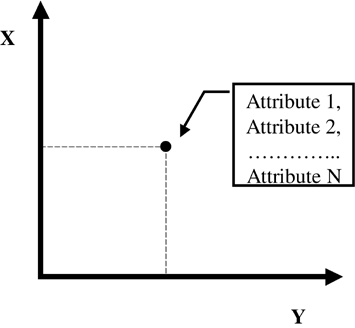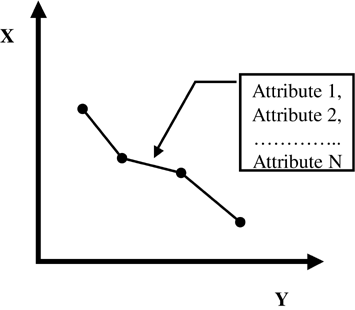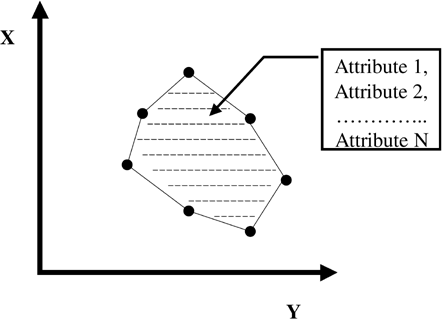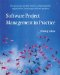# Geographical Data Types

To understand how map databases store information, it is important to understand the basic geographic data types used to represent real-world geographic phenomena. In the vector data model, geographic data is stored in discrete points, lines, and polygons. Points, lines, and polygons are respectively zero-, one-, and two-dimensional static representations of real-world phenomena in terms of simple x, y coordinates.

#### Points

Points refer to objects that have location and attribute information, but are not large enough to be represented as areas (see Figure 4.6). Whether an object is represented as a point or a polygon depends on the level of abstraction and scale of the map. Cities that would be represented as points in a country-level map might be represented as polygons in a regional map. Other examples of points include points of interest (POIs) and parcel centroids, such as postal codes or addresses. Points have no dimensions.

##### Figure 4.6. Point Primitive.#### Nodes

Nodes are a special type of point that represent a junction or the endpoint of a line. Nodes are the same as points in all other respects. Nodes provide information that includes connectivity between lines and information about adjacent polygons and enclosed islands.

#### Lines and Arcs

Lines are one-dimensional objects that have length but no area (see Figure 4.7). Lines must begin and end with a node. In the case of a road, a line will represent the street center line. The actual width of a road will be an attribute of the line. Other attributes of a road might include street name , address range, speed, and direction of travel. At the country level, a line is an adequate representation of a road. At the regional level, a line might not be adequate and a road would be represented using a polygon area of paving. Lines might also be used to represent rivers and railways. An arc is a multisegment line.

##### Figure 4.7. Line Primitive.#### Polygons

The simplest definition of a polygon is a set of connected lines that form a closed mathematical figure (see Figure 4.8). Polygons can have any number of points and can be any shape or size. Other ways to represent a polygon include the set of x, y coordinates that form its boundary or the area contained by the boundary. Polygons can have holes, contain other polygons, and be directly adjacent to other polygons.

##### Figure 4.8. Polygon Primitive.Each geographic primitive has attached information that describes it. In mobile location services, the most important attributes relate to the road network, such as speed, street names , turn restrictions, and connectivity.

#### Computer Storage of Geographic Data Structures

Now that we have developed a conceptual notion of our map using points, lines, and polygons, we must consider how this data is stored in a computer. The method used to store this data has a large impact on how large the map database is and how quickly data can be retrieved and used in a mobile location services application.

Simple spatial entities have the following characteristics: points will have an (x, y) coordinate, a simple line with only two nodes will have two (x, y) coordinates, an arc will have n number of (x, y) coordinates, and a polygon could have either n number of lines or n number of points. To achieve sufficient precision, it is necessary to use a 32-bit or 64-bit real data type for each value in the coordinate pair. Thus, because there are 8 bits in a byte, a point would require 8 or 16 bytes of storage, a line requires a minimum of 16 bytes of storage, and a polygon requires a minimum of 24 bytes. Because map databases can contain many millions of spatial entities and each can have linked attributes, it is easy to see how a map database can become very large.Software Project Management in Practice
ISBN: 0201737213
EAN: 2147483647
Year: 2005
Pages: 150
Authors: Pankaj Jalote

Similar book on Amazon